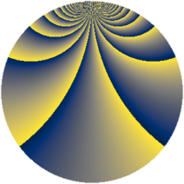# Properties

 Label 912.2.cpLevel $912$ Weight $2$ Character orbit 912.cp Rep. character $\chi_{912}(35,\cdot)$ Character field $\Q(\zeta_{36})$ Dimension $1872$ Newform subspaces $1$ Sturm bound $320$ Trace bound $0$

# Related objects

## Defining parameters

 Level: $$N$$ $$=$$ $$912 = 2^{4} \cdot 3 \cdot 19$$ Weight: $$k$$ $$=$$ $$2$$ Character orbit: $$[\chi]$$ $$=$$ 912.cp (of order $$36$$ and degree $$12$$) Character conductor: $$\operatorname{cond}(\chi)$$ $$=$$ $$912$$ Character field: $$\Q(\zeta_{36})$$ Newform subspaces: $$1$$ Sturm bound: $$320$$ Trace bound: $$0$$

## Dimensions

The following table gives the dimensions of various subspaces of $$M_{2}(912, [\chi])$$.

Total New Old
Modular forms 1968 1968 0
Cusp forms 1872 1872 0
Eisenstein series 96 96 0

## Trace form

 $$1872q - 12q^{3} - 24q^{4} - 12q^{6} - 24q^{7} + O(q^{10})$$ $$1872q - 12q^{3} - 24q^{4} - 12q^{6} - 24q^{7} - 36q^{10} - 6q^{12} - 24q^{13} - 36q^{16} - 24q^{18} - 24q^{19} + 6q^{21} - 24q^{22} - 12q^{24} - 6q^{27} + 24q^{28} + 36q^{30} - 24q^{33} - 24q^{34} - 12q^{36} - 48q^{37} - 48q^{39} - 24q^{40} - 96q^{42} - 24q^{43} - 6q^{45} - 12q^{46} - 12q^{48} - 816q^{49} - 12q^{51} + 24q^{52} + 54q^{54} - 48q^{55} - 48q^{58} + 30q^{60} - 24q^{61} - 12q^{64} + 48q^{66} - 24q^{67} - 6q^{69} - 12q^{70} - 48q^{72} + 144q^{75} + 24q^{76} + 12q^{78} - 24q^{81} - 24q^{82} + 78q^{84} + 72q^{85} - 12q^{87} - 12q^{88} + 96q^{90} + 60q^{91} - 30q^{93} - 48q^{94} + 276q^{96} - 48q^{97} + 42q^{99} + O(q^{100})$$

## Decomposition of $$S_{2}^{\mathrm{new}}(912, [\chi])$$ into newform subspaces

Label Dim. $$A$$ Field CM Traces $q$-expansion
$$a_2$$ $$a_3$$ $$a_5$$ $$a_7$$
912.2.cp.a $$1872$$ $$7.282$$ None $$0$$ $$-12$$ $$0$$ $$-24$$